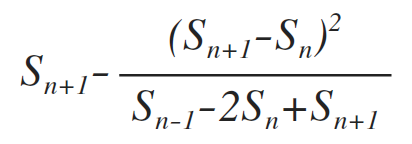# Java 8 新特性：全新的 Stream API

Java 8 引入了全新的 Stream API。这里的 Stream 和 I/O 流不同，它更像具有 Iterable 的集合类，但行为和集合类又有所不同。

Stream API 引入的目的在于弥补 Java 函数式编程的缺陷。对于很多支持函数式编程的语言，map()、reduce() 基本上都内置到语言的标准库中了，不过，Java 8 的 Stream API 总体来讲仍然是非常完善和强大，足以用很少的代码完成许多复杂的功能。

public static void main(String[] args) {
List<Integer> numbers = Arrays.asList(1, 2, 3, 4, 5, 6, 7, 8, 9, 10);
Stream<Integer> stream = numbers.stream();
stream.filter((x) -> {
return x % 2 == 0;
}).map((x) -> {
return x * x;
}).forEach(System.out::println);
}


class NaturalSupplier implements Supplier<Long> {

long value = 0;

public Long get() {
this.value = this.value + 1;
return this.value;
}
}


public static void main(String[] args) {
Stream<Long> natural = Stream.generate(new NaturalSupplier());
natural.map((x) -> {
return x * x;
}).limit(10).forEach(System.out::println);
}


class FibonacciSupplier implements Supplier<Long> {

long a = 0;
long b = 1;

@Override
public Long get() {
long x = a + b;
a = b;
b = x;
return a;
}
}

public class FibonacciStream {

public static void main(String[] args) {
Stream<Long> fibonacci = Stream.generate(new FibonacciSupplier());
fibonacci.limit(10).forEach(System.out::println);
}
}


List<Long> list = fibonacci.skip(20).limit(10).collect(Collectors.toList());


π/4 = 1 - 1/3 + 1/5 - 1/7 + 1/9 - ...


class PiSupplier implements Supplier<Double> {

double sum = 0.0;
double current = 1.0;
boolean sign = true;

@Override
public Double get() {
sum += (sign ? 4 : -4) / this.current;
this.current = this.current + 2.0;
this.sign = ! this.sign;
return sum;
}
}

Stream<Double> piStream = Stream.generate(new PiSupplier());
piStream.skip(100).limit(10)
.forEach(System.out::println);


3.1514934010709914
3.1317889675734545
3.1513011626954057
3.131977491197821
3.1511162471786824
3.1321589012071183
3.150938243930123
3.132333592767332
3.1507667724908344
3.1325019323081857class EulerTransform implements Function<Double, Double> {

double n1 = 0.0;
double n2 = 0.0;
double n3 = 0.0;

@Override
public Double apply(Double t) {
n1 = n2;
n2 = n3;
n3 = t;
if (n1 == 0.0) {
return 0.0;
}
return calc();
}

double calc() {
double d = n3 - n2;
return n3 - d * d / (n1 - 2 * n2 + n3);
}
}

Stream<Double> piStream2 = Stream.generate(new PiSupplier());
piStream2.map(new EulerTransform())
.limit(10)
.forEach(System.out::println);


0.0
0.0
3.166666666666667
3.1333333333333337
3.1452380952380956
3.13968253968254
3.1427128427128435
3.1408813408813416
3.142071817071818
3.1412548236077655


Stream<Double> piStream3 = Stream.generate(new PiSupplier());
piStream3.map(new EulerTransform())
.map(new EulerTransform())
.map(new EulerTransform())
.map(new EulerTransform())
.map(new EulerTransform())
.limit(20)
.forEach(System.out::println);


20 项之内可以计算出极其精确的值：

...
3.14159265359053
3.1415926535894667
3.141592653589949
3.141592653589719